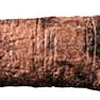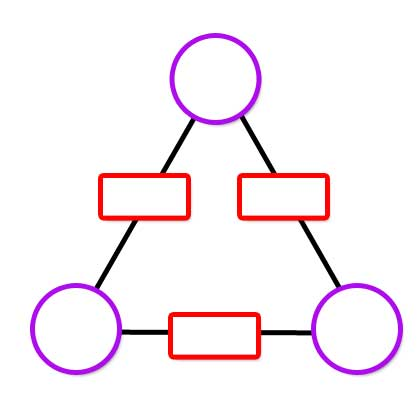#### You may also like### Where Can We Visit?

Charlie and Abi put a counter on 42. They wondered if they could visit all the other numbers on their 1-100 board, moving the counter using just these two operations: x2 and -5. What do you think?### Babylon Numbers

Can you make a hypothesis to explain these ancient numbers?### Ishango Bone

Can you decode the mysterious markings on this ancient bone tool?

# Arithmagons

##### Age 11 to 16 Challenge Level:

An arithmagon is a polygon with numbers at its vertices which determine the numbers written on its edges.

Check you understand how the values at the vertices determine the values on the edges in the arithmagons generated by the interactivity:If you are given the values on the edges, can you find a way to work out what values belong at the vertices?
Use the interactivity below to test out your strategies.
There are four different challenge levels to try.Once you are confident that you can work out the values at the vertices efficiently, here are some questions you might like to consider:

• Can you describe a strategy to work out the values at the vertices irrespective of the values given for the edges?

• By looking at the numbers on the edges, how can you predict whether the numbers at the vertices will all be positive?

• By looking at the numbers on the edges, how can you predict whether the numbers at the vertices will all be whole numbers?

• What happens to the numbers at the vertices if you increase one or more of the numbers on the edges by 1? By 2? ... By n?

• What relationships can you find in square arithmagons? Or pentagonal arithmagons?

A poster of this resource is available here.